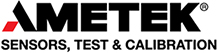Skip to content

# What is Tensile StrengthTensile strength refers to the ability of a structure to resist loads without failure because of excessive stress or deformation.

Tensile strength is often referred to as ultimate tensile strength and is calculated by dividing the peak tension force the sample withstands by its cross sectional area.

A tensile tester is used to measure tensile strength. A load cell is fitted to the tensile tester to measure tensile force. In addition a tensile test is commonly used to measure other properties of materials such as Young’s modulus of elasticity, yield stress, elongation, strain and ultimate tensile stress.